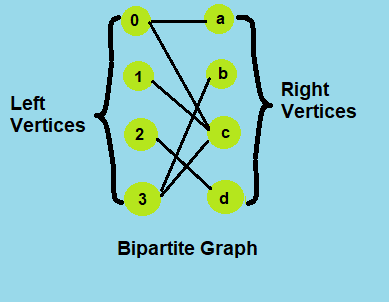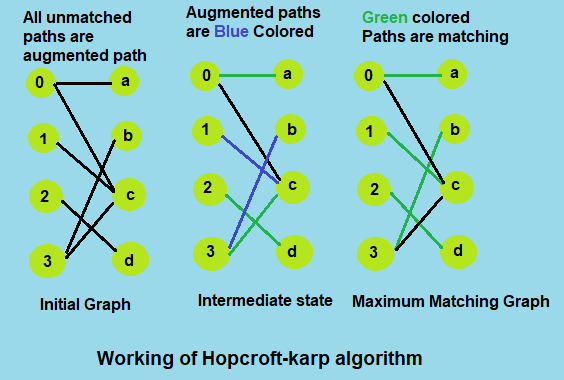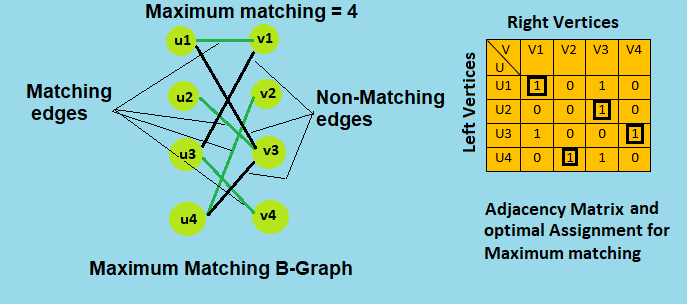# Understanding pairing nodes in Graphs (Maximum Matching)

The problem of pairing nodes in the graph may seem to be an easy task but turns out to be a difficult task if not approached in the correct way. Moreover, this problem has huge applications in resource allocation and optimization problems and hence, understanding the concepts behind this and being able to visualize a problem in a graph representation opens up a new understanding and approach. In this article, we will explore the basic concepts behind it, ideas behind three efficient algorithms to tackle this and some real life applications.

Given a graph G = (V,E), a matching M in G is a set of pairwise non-adjacent edges, none of which are loops; that is, no two edges share a common vertex. A vertex is matched (or saturated) if it is an endpoint of one of the edges in the matching. Otherwise the vertex is unmatched.

Matching and Not-Matching edges Given a matching M, edges that are part of matching are called Matching edges and edges that are not part of M (or connect free nodes) are called Not-Matching edges.

Maximum Matching A maximal matching is a matching M of a graph G with the property that if any edge not in M is added to M, it is no longer a matching, that is, M is maximal if it is not a subset of any other matching in graph G. In other words, a matching M of a graph G is maximal if every edge in G has a non-empty intersection with at least one edge in M.

Alternate Path An alternating path is a path that begins with an unmatched vertex and whose edges Belong alternately to the matching and not to the matching.

Augmented Path An augmenting path is an alternating path that starts from and ends on free (unmatched) vertices.

Bipartite Graph A bipartite graph (or bigraph) is a graph whose vertices can be divided into two disjoint and independent sets U and V such that every edge connects a vertex in U to one in V. Vertex sets U and V are usually called the parts of the graph. Equivalently, a bipartite graph is a graph that does not contain any odd-length cycles.Here we will see three algorithms for finding Maximum Matching in a graph:

• Hopcroft-Karp Algorithm
• Hungarian Algorithm
• Blossoms' Algorithm

# Hopcroft-Karp Algorithm

The Hopcroft Karp algorithm is based on below concept.

A matching M is not maximum if there exists an augmenting path. It is also true other way, i.e, a matching is maximum if no augmenting path exists.

So the idea is to one by one look for augmenting paths. And add the found paths to current matching.

The Hopcroft–Karp algorithm repeatedly increases the size of a partial matching by finding augmenting paths. Sequences of edges that alternate between being in and out of the matching, such that swapping which edges of the path are in and which are out of the matching produces a larger matching. However, instead of finding just a single augmenting path per iteration, the algorithm finds a maximal set of shortest augmenting paths. As a result, O(√V) iterations are needed. As BFS or DFS takes O(V + E) (i.e O(E) as E > V in genral) time per iteration, so overall time complexity of algorithm is O(E√V), which can be O(V3/2) for the dense graph in the worst case.The Hopcroft-Carp Algorithm only works for Bipartite Graphs and not for any general graph.

# Hungarian Algorithm

The Hungarian algorithm can be executed by manipulating the weights of the bipartite graph in order to find a stable, maximum (or minimum) weight matching. This can be done by finding a feasible labeling of a graph that is perfectly matched, where a perfect matching is denoted as every vertex having exactly one edge of the matching.

The idea is to make a complete graph from the given bipartite graph by simply adding edges of weight `0` (maxinum weight matching) and then convert it to assignment problem and then solve it for a perfect matching.

In each step of the algorithm's loop we will either be increasing the size of M or Eℓ so this process must terminate. Furthermore, when the process terminates, M will be a perfect matching in Eℓ for some feasible labeling ℓ. So, by the Kuhn-Munkres theorem (If ℓ is feasible and M is a Perfect matching in Eℓ then M is a max-weight matching), M will be a max-weight matching.

In each phase of algorithm, M increases by 1 so there are at most V phases and O(V2) work per phase is done so the total running time is O(V3).So, Hungarian Algorithm is for weighted Bipartite graph, it can also work for non-weighted Bipartite graph by simply assuming each edge is of equal weight say 1.

# Blossoms' Algorithm

The goal of the algorithm is to shrinks a blossom into a single node in order to reveal augmenting paths. It works by running the Hungarian algorithm until it runs into a blossom, which it then shrinks down into a single vertex. Then, it begins the Hungarian algorithm again. If another blossom is found, it shrinks the blossom and starts the Hungarian algorithm, and so on.

Overall running time of Blossoms' algorithm is O(E*V2), which is can be O(V4) for dense graph in the worst case.Blossoms' algorithm works for any general graph, i.e. it works for bipartite graphs as well as the graphs with odd length cycles. But there is no meaning in applying Blossoms' algorithm on a bipartite graph as the whole concept of the algorithm is based on blossom contraction (odd length cycles contraction) and which is not present in the bipartite graph.